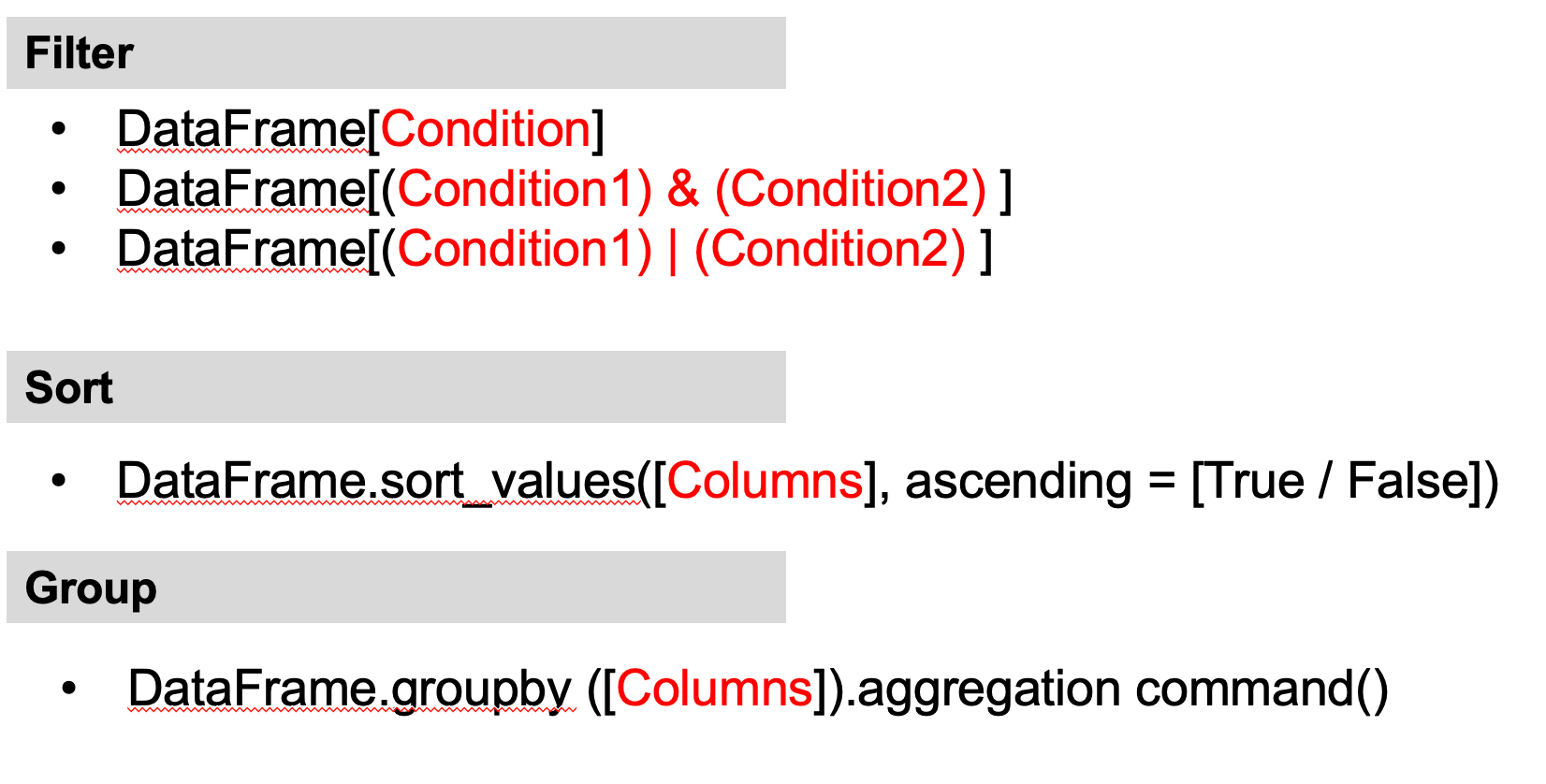# Data Analytics Part-3

## Slice data using simple conditions

You can also slice data using conditions based on the value of one or more columns.

The structure of the command line is: DataFrame[Condition]

### Example 1: Display all the rows where "Specialisation" is "Health and Medicine"

Condition: data["Specialisation"] == "Health and Medicine" the code is:
```	    data[data["Specialisation"] == "Health and Medicine"]

```## Slice data using simple conditions

### Example: Display all the rows where "Enrolled _ UnderGraduate" is 1000 and above

```	    data[data["Enrolled _ UnderGraduate"] >= 1000]

```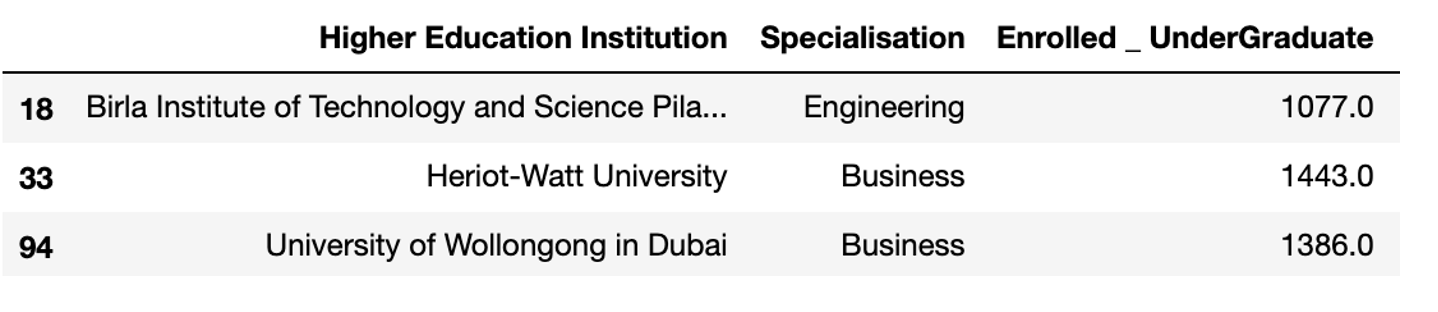### Example: Display total number of rows where "Enrolled _ UnderGraduate" is greater or equal to the average "Enrolled _ UnderGraduate"

```	    data[data["Enrolled _ UnderGraduate"] >= data["Enrolled _ UnderGraduate"].mean()]

```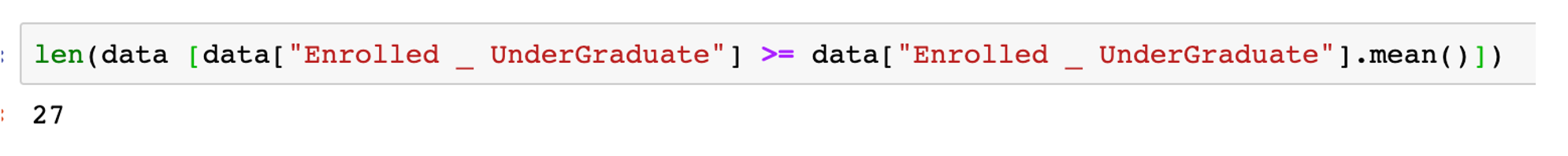## Slice data using multiple conditions

To slice data using more than one condition, you need to put each condition inside a bracket ( ). You also need to use a logical operator. The symbol & is used for and. The symbol | is used for or.

The structure of the command line is:

• DataFrame[(Condition) & (Condition)]
• DataFrame[(Condition) | (Condition)]

### Example: Display all the rows where "Specialisation" is "Law" or "Humanities"

```        (data["Specialisation"] == "Law") | (data["Specialisation"] == "Humanities")
```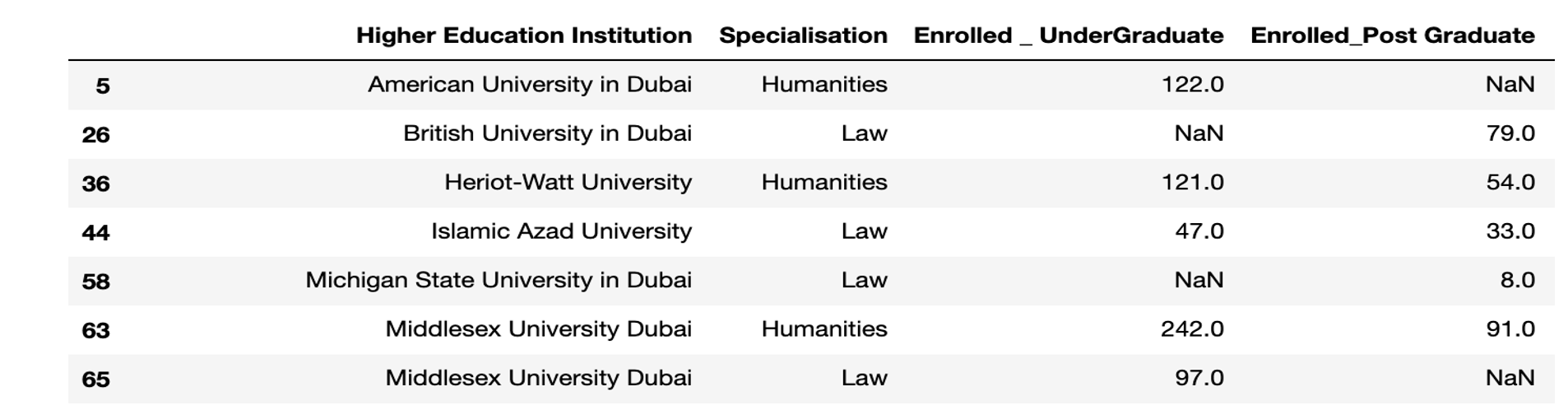### Example2: Display all the rows where " Enrolled _ UnderGraduate" value is from 500 to 800

```        (data[Enrolled _ UnderGraduate"] >= 500) & (data["Enrolled _ UnderGraduate"] <= 800)
```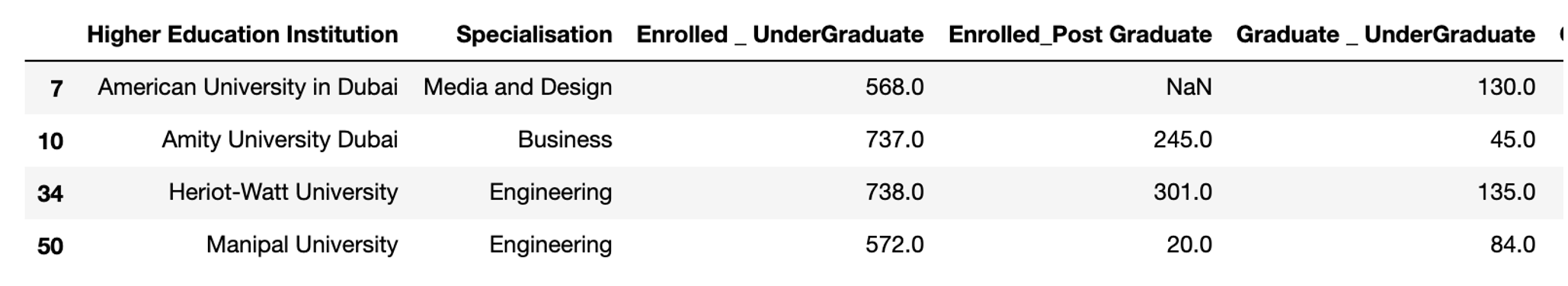## Sorting data with one column

Sorting data is a technique that display data in an ascending or descending order.

```        data.sort_values(['CoulmnName'], ascending=False)
```

By default, the data will be sorted in ascending order.

### Example: Sort the Enrolled _ UnderGraduate column

```        data.sort_values('Enrolled _ UnderGraduate')
```
```        data.sort_values('Enrolled _ UnderGraduate', ascending = False)
```## Sorting data with more than one column

To sort data based on more than one column, you need to include all columns inside the square bracket.

### Example: Sort Specialisation in ascending order, Enrolled undergraduate in descending order

```        data.sort_values(['Specialisation','Enrolled _ UnderGraduate'],ascending=[True,False])
```## Grouping Data

Grouping data by columns is used when you have duplicated data in a particular column. For example you, the same student is doing more than one course and has grades in each course. The function needed for this operation is .groupby

```        dataframe.groupby(['Column1', 'Column2']).mean()
```

Instead of mean(), you can use sum(), min() or any of the aggregation commands.

### Example: Display the total Number of students enrolled in each specialisation

```        data.groupby('Specialization') [ ['Total Enrolled']].sum()
```### Example 2: Total Number of students enrolled in each specialisation, by each Institution

```        data.groupby(['Higher Education Institution', 'Specialization')] [ ['Total Enrolled']].sum()
```## Summary of Pandas Commands